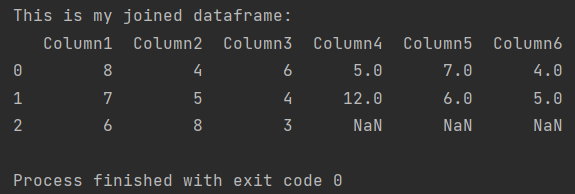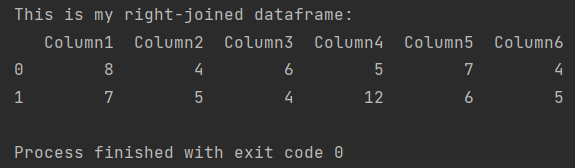Pandas How To Uncategorized How to join two dataframes on index

# How to join two dataframes on index

In this post you will learn how to join two dataframes on index in Pandas.

Joining dataframes in Pandas is easy because Pandas offers several functions that have extensive parameters. Dataframe merging in Pandas is a very common activity, so it’s worth learning how to merging dataframes in Pandas in different ways.

In this post, I will focus on the use of the join function, which allows you to connect a dataframe using several parameters.

## How to join two dataframes on index in Pandas

The join function offers many parameters, but I will first show you how to connect a dataframe on index in the simplest way.

These are my example dataframes that I want to combine on index.

Note that the dataframes are of different sizes. The first one contains three values in each column. The second dataframe contains only two values. It is very important how you connect the dataframes, because this will affect which values will be merged into the connected dataframe.

```import pandas as pd

my_df1 = pd.DataFrame({'Column1': [8, 7, 6],
'Column2': [4, 5, 8],
'Column3': [6, 4, 3]})

my_df2 = pd.DataFrame({'Column4': [5, 12],
'Column5': [7, 6],
'Column6': [4, 5]})

```

This is the sample code of the join function without using additional parameters.

```import pandas as pd

my_df1 = pd.DataFrame({'Column1': [8, 7, 6],
'Column2': [4, 5, 8],
'Column3': [6, 4, 3]})

my_df2 = pd.DataFrame({'Column4': [5, 12],
'Column5': [7, 6],
'Column6': [4, 5]})

my_df = my_df1.join(my_df2)

print(f'This is my joined dataframe: \n{my_df}')

```The combined dataframe contains all the values from both dataframes. Pandas replaced the missing values with NaN (not a number).

Pandas offers four different methods of combining dataframes:
– left (default value) – dataframe on the left side + part in common with the dataframe on the right side
– outer – joins all data and inserts NaN values in the missing places (same effect as above)
– right – dataframe on the right side + common part with the dataframe on the left side
– inner – only the common part of dataframes

In this case, left and outer will have exactly the same effect as above. Let’s see the other methods.

### How to right-join two dataframes on index in Pandas

Below I will use the right-join method. As a parameter of the join function, I will add how and enter the method type.

```import pandas as pd

my_df1 = pd.DataFrame({'Column1': [8, 7, 6],
'Column2': [4, 5, 8],
'Column3': [6, 4, 3]})

my_df2 = pd.DataFrame({'Column4': [5, 12],
'Column5': [7, 6],
'Column6': [4, 5]})

my_df = my_df1.join(my_df2, how='right')

print(f'This is my right-joined dataframe: \n{my_df}')

```As you can see some data has disappeared. Pandas displayed the entire dataframe on the right and only the matching part of the dataframe on the left.

In this case, inner-join will give the same result because there is no mismatching data in the dataframe on the right.

Documentation of join function
How to merge two dataframes

Tags:

## How to resolve ValueError: Index has duplicate keys error?How to resolve ValueError: Index has duplicate keys error?

In this post, you will learn how to solve the “ValueError: Index has duplicate keys” error in Pandas.

## How to rename columnsHow to rename columns

Learn all the possibilities of how to rename columns in Pandas and choose the most convenient one for you.

## How to calculate cumulative sum in PandasHow to calculate cumulative sum in Pandas

Here’s how to calculate cumulative sum in Pandas.